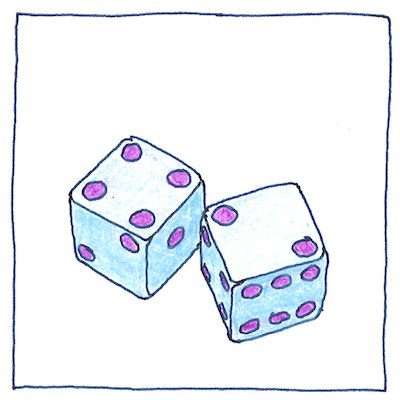# 1654

## The book of science

Tom Sharp

 Blaise Pascal, Pierre de Fermat probability

## Probability theory

• Pascal and Fermat corresponded
• over “the problem of points,” that is,
• how to fairly divide the stakes of a game
• if the game were left unfinished.
• They were the first to realize
• that fairness depends on the number
• of possible outcomes left in the game.
• Fermat drew a tree of all possible outcomes,
• and counted how many outcomes
• led to each player winning.
• Pascal showed how to calculate the number of outcomes
• and introduced the notion of expected value,
• the weighted average of all possible outcomes.

## Odds

• One’s odds are the ratio
• of the probability of an event happening
• to the probability of it not happening.
• You might have a one in five odds
• given that you noticed it at all.

## Betting

• My advice: Retire early and beat the odds.
• the company should end up paying you to live longer;
• however, the law of large numbers is against you
• and they are banking on that. Actuarial tables say
• you will die before you’re eighty-five.
• Go ahead and defy them, and don’t forget
• quality is as important as quantity. Defy them; live a long and happy life.
• You’ll be in trouble only if everyone does it.

This correspondence between Pascal and Fermat influenced Christiaan Huygens, who published the first book on probability theory three years later. Huygens was the guy who first declared that Saturn has a ring, and who invented the pendulum clock.

Fermat was the first to evaluate the integral of general power functions, which influenced both Newton and Leibnitz in their creation of calculus.